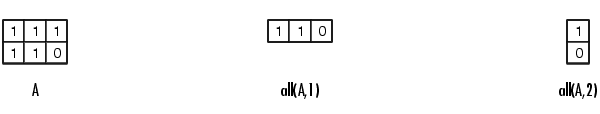MATLAB Function Referenceall

Determine if all array elements are nonzero

Syntax

• ```B = all(A)
B = all(A,` dim`)
```

Description

```B = all(A) ``` tests whether all the elements along various dimensions of an array are nonzero or logical `1` (`true`).

If `A` is a vector, `all(A)` returns logical `1` (`true`) if all the elements are nonzero and returns logical `0` (`false`) if one or more elements are zero.

If `A` is a matrix, `all(A)` treats the columns of `A` as vectors, returning a row vector of logical `1`'s and `0`'s.

If `A` is a multidimensional array, `all(A)` treats the values along the first nonsingleton dimension as vectors, returning a logical condition for each vector.

```B = all(A, dim) ``` tests along the dimension of `A` specified by scalar `dim`.Examples

Given

• ```A = [0.53 0.67 0.01 0.38 0.07 0.42 0.69]
```

then `B = (A < 0.5)` returns logical `1` (`true`) only where `A` is less than one half:

• ```0   0   1   1   1   1   0
```

The `all` function reduces such a vector of logical conditions to a single condition. In this case, `all(B)` yields `0`.

This makes `all` particularly useful in `if` statements:

• ```if all(A < 0.5)
```do something
```end
```

where code is executed depending on a single condition, not a vector of possibly conflicting conditions.

Applying the `all` function twice to a matrix, as in `all(all(A))`, always reduces it to a scalar condition.

• ```all(all(eye(3)))
ans =
0
```

See Also

`any`, logical operators (elementwise and short-circuit), relational operators, `colon`

Other functions that collapse an array's dimensions include `max`, `mean`, `median`, `min`, `prod`, `std`, `sum`, and `trapz`.

© 1994-2005 The MathWorks, Inc.